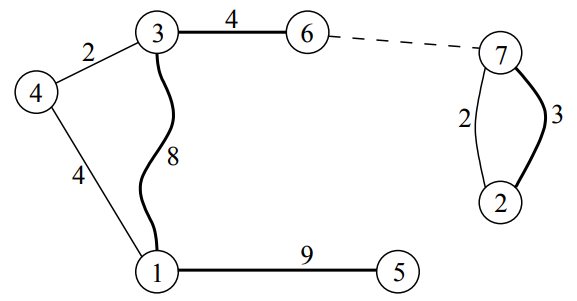# P4381 [IOI2008]Island

• 505通过
• 2.1K提交
• 题目提供者 goodbye2018
• 评测方式 云端评测
• 标签 动态规划,动规,dp 树的直径 队列 IOI 2008 O2优化 新云端 高性能
• 难度 NOI/NOI+/CTSC
• 时空限制 1000ms / 256MB
• 提示：收藏到任务计划后，可在首页查看。

## 题目描述

你准备浏览一个公园，该公园由 $N$ 个岛屿组成，当地管理部门从每个岛屿 $i$ 出发向另外一个岛屿建了一座长度为 $L_i$ 的桥，不过桥是可以双向行走的。同时，每对岛屿之间都有一艘专用的往来两岛之间的渡船。相对于乘船而言，你更喜欢步行。你希望经过的桥的总长度尽可能长，但受到以下的限制：

• 可以自行挑选一个岛开始游览。
• 任何一个岛都不能游览一次以上。
• 无论任何时间，你都可以由当前所在的岛 $S$ 去另一个从未到过的岛 $D$。从 $S$ 到 $D$ 有如下方法：
• 步行：仅当两个岛之间有一座桥时才有可能。对于这种情况，桥的长度会累加到你步行的总距离中。
• 渡船：你可以选择这种方法，仅当没有任何桥和以前使用过的渡船的组合可以由 $S$ 走到 $D$ (当检查是否可到达时，你应该考虑所有的路径，包括经过你曾游览过的那些岛)。

注意，你不必游览所有的岛，也可能无法走完所有的桥。

请你编写一个程序，给定 $N$ 座桥以及它们的长度，按照上述的规则，计算你可以走过的桥的长度之和的最大值。

## 输入输出格式

输入格式：

第一行包含 $N$ 个整数，即公园内岛屿的数目。

随后的 $N$ 行每一行用来表示一个岛。第 $i$ 行由两个以单空格分隔的整数，表示由岛 $i$ 筑的桥。第一个整数表示桥另一端的岛，第二个整数表示该桥的长度 $L_i$。你可以假设对于每座桥，其端点总是位于不同的岛上。

输出格式：

仅包含一个整数，即可能的最大步行距离。

## 输入输出样例

输入样例#1： 复制
7
3 8
7 2
4 2
1 4
1 9
3 4
2 3
输出样例#1： 复制
24

## 说明

样例解释样例 $N=7$ 座桥，分别为 $(1-3), (2-7), (3-4), (4-1), (5-1), (6-3)$ 以及 $(7-2)$。注意连接岛 $2$ 与岛 $7$ 之间有两座不同的桥。

其中一个可以取得最大的步行距离的方法如下：

• 由岛 $5$ 开始。
• 步行长度为 $9$ 的桥到岛 $1$。
• 步行长度为 $8$ 的桥到岛 $3$。
• 步行长度为 $4$ 的桥到岛 $6$。
• 搭渡船由岛 $6$ 到岛 $7$。
• 步行长度为 $3$ 的桥到岛 $2$。

最后，你到达岛 $2$，而你的总步行距离为 $9+8+4+3=24$。

只有岛 $4$ 没有去。注意，上述游览结束时，你不能再游览这个岛。更准确地说：

• 你不可以步行去游览，因为没有桥连接岛 $2$ (你现在的岛) 与岛 $4$。
• 你不可以搭渡船去游览，因为你可由当前所在的岛 $2$ 到达岛 $4$。一个方法是：走 $(2-7)$ 桥，再搭你曾搭过的渡船由岛 $7$ 去岛 $6$，然后走 $(6-3)$ 桥，最后走 $(3-4)$ 桥。

数据范围

对于 $100\%$ 的数据，$2\leqslant N\leqslant 10^6,1\leqslant L_i\leqslant 10^8$。

提示
标程仅供做题后或实在无思路时参考。
请自觉、自律地使用该功能并请对自己的学习负责。
如果发现恶意抄袭标程，将按照I类违反进行处理。